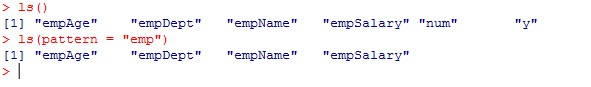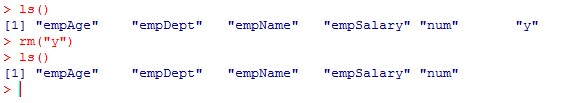# R Variables

In this tutorial you will learn about the R Variables and its application with practical example.

## R Variables

Variables is an identifier used to refer memory location in computer memory that holds a value for that variable, this value can be changed during the execution of the program. When you create a variable in R, this means you are allocating some space in the memory for that variable. The size of memory block allocated and type of the value it holds is completely dependent upon the type of variable. In R, a variable can be used to hold a number, a string, an object, a statistical result, vector, dataset, a model prediction etc.

## Rules for naming a variable –

Constant and variable names cannot contain whitespace characters, mathematical symbols, arrows, private-use (or invalid) Unicode code points, or line- and box-drawing characters.

• Variable names can be a combination of letters, numbers, period (.) and underscore (_).
• Keywords are not allowed to be used as a variable name.
• Blank spaces are not allowed in variable name.
• Variable names must start with a letter or a period, however if it starts with a period, it cannot be followed by a digit.

Recommendation :- Variable name must be readable and should be relative to its purpose.

Example:- Valid Variable names in R

Example:- Invalid Variable names in R

## Declaring Variables In R

In R, variables can be created by assigning it value either using leftward (<-) or equal (=) operator.

Syntax:-

Example:-

Output:-

## Listing Workspace Variables

In R, ls() function is used to print all the variables available in current workspace, we can also pass patterns to find the variable names.

Example:-## Deleting Variables

In R, rm() function is used to remove a variables from current workspace.

Syntax:-

Here, varName is replaced with variable name.

Example:-In this tutorial we have learn about the R Variables and its application with practical example. I hope you will like this tutorial.